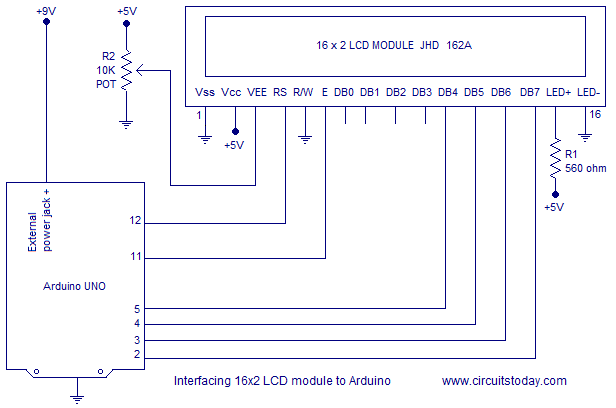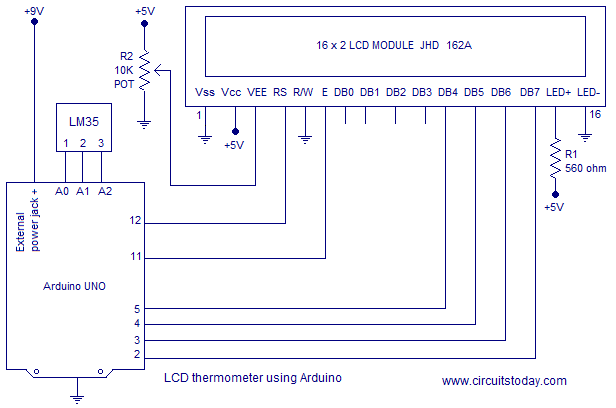# Interfacing LCD to Arduino uno

LCD modules form a very important part in many arduino based embedded system designs. So the knowledge on interfacing LCD to arduino is very essential in designing  embedded systems. This article is about interfacing a 16×2 LCD  to Arduino. JHD162A is the LCD module used here. JHD162A is a 16×2 LCD module based on the HD44780 driver from Hitachi. The JHD162A has 16 pins and can be operated in 4-bit mode or 8-bit mode. Here we are using the LCD module in 4-bit mode. First, I will show you how to display plain text messages on the LCD module using arduino and then  few useful projects using LCD and arduino. Before going in to the details of the project, let’s have a look at the JHD162A LCD module.

### JHD162A LCD module 16×2.

The JHD162A has 16 pins and can be operated in 4-bit mode or 8-bit mode. Here we are using the LCD module in 4-bit mode. Before going in to the details of the project, let’s have a look at the JHD162A LCD module.The schematic of a JHD162A LCD module is given below.
Pin1(Vss):Ground pin of the LCD module.
Pin2(Vcc):+5V supply is given to this pin
Pin3(VEE):Contrast adjustment pin. This is done by connecting the ends of a 10K potentimeter to +5V and ground and then connecting the slider pin to the VEE pin. The voltage at the VEE pin defines the contrast. The normal setting is between 0.4 and 0.9V.
Pin4(RS):Register select pin.The JHD162A has two registers namely command register and data register. Logic HIGH at RS pin selects data register and logic LOW at RS pin will select command register. If we make the RS pin HIGH and put a data on the data lines (DB0 to DB7) it will be recognized as a data. If we make the RS pin LOW and put a data on the data lines, then it will be taken as a command.
Pin5(R/W): Read/Write modes. This pin is used for selecting between read and write modes. Logic HIGH at this pin activates read mode and logic LOW at this pin activates write mode.
Pin6(E): This pin is meant for enabling the LCD module. A HIGH to LOW signal at this pin will enable the module.
Pin7(DB0) to Pin14(DB7):  These are data pins. The commands and data are put on these pins.
Pin15(LED+): Anode of the back light LED. When operated on 5V, a 560 ohm resistor should be connected in series to this pin. In arduino based projects the back light LED can be powered from the 3.3V source on the arduino board.
Pin16(LED-): Cathode of the back light LED.
For knowing more about LCD module JHD162A and it interfacing, read this article: Interfacing 16×2 LCD and 8051 microcontroller. The circuit diagram of interfacing LCD to arduino for displaying a text message is shown below.

#### Circuit diagram.RS pin of the LCD module is connected to digital pin 12 of the arduino. R/W pin of the LCD is grounded. Enable pin of the LCD module is connected to digital pin 11 of the arduino. In this project, the LCD module and arduino are interfaced in the 4-bit mode. That means only four of the digital input lines( DB4 to DB7 of the LCD are used). This method is very simple, requires less connections and you can almost utilize the full potential of the LCD module. Digital lines DB4, DB5, DB6 and DB7 are interfaced to digital pins 5, 4, 3 and 2 of the Arduino. The 10K potentiometer is used for adjusting the contrast of the display. 560 ohm resistor R1 limits the current through the back light LED. The arduino can be powered through the external power jack provided on the board. +5V required in some other parts of the circuit can be tapped from the 5V source on the arduino board. The arduino can be also powered from the PC through the USB port. The full program for interfacing LCD to arduino is shown below.

#### Program.

```#include<LiquidCrystal.h>

LiquidCrystal lcd(12, 11, 5, 4, 3, 2);  // sets the interfacing pins

void setup()
{
lcd.begin(16, 2);  // initializes the 16x2 LCD
}

void loop()
{
lcd.setCursor(0,0);           //sets the cursor at row 0 column 0
lcd.print("16x2 LCD MODULE"); // prints 16x2 LCD MODULE
lcd.setCursor(2,1);           //sets the cursor at row 1 column 2
lcd.print("HELLO WORLD");     // prints HELLO WORLD
}```

Library function “LiquidCrystal.h” is used for displaying the desired characters on the LCD module. It is readily available with the Arduino user interface and it can be accessed through the “Import library” in the “sketch” tab in the main menu bar. The LiquidCrystal.h provides functions for almost all applications like printing a string, setting the cursor, initializing the LCD, scrolling the display etc.
##### Program for scrolling the display.
A simple program for scrolling a text on the LCD using arduino is shown here. This is done using the “scroll()” function. For example the function “lcd.scrollDisplayRight()” will scroll the display to right and the function”lcd.scrollDisplayLeft()” will scroll the display to left. A “for” loop is used for selecting the number of positions to scroll at a time. In the program shown below, it is chosen to be 2 because the text to be displayed is comparatively long. For shorter texts more number of positions must be scrolled at a time to get a smooth display.
```#include <LiquidCrystal.h>
int i=0;

LiquidCrystal lcd(12, 11, 5, 4, 3, 2);

void setup()
{
lcd.begin(16, 2);                              //initializes 16x2 LCD
lcd.print("16x2 LCD MODULE JHD162A & ARDUINO-UNO"); //text to display
}

void loop()
{
for(i=0;i<2;i++)
{lcd.scrollDisplayLeft();}   //scrolls display left by two positions
delay(800);                  //sets the speed at which display moves
}```

#### Digital thermometer with LCD display using arduino.

This is just a practical implementation of the interfacing of LCD and Arduino. A simple digital thermometer using arduino and 3 digit seven segment display had been already published here. You can find that article here: Digital thermometer using arduino. Read this article before attempting the LCD version. LM35 is the temperature sensor used in this project. It is a three terminal linear analog temperature sensor. The output voltage of the LM35 increases 10mV per °C rise in temperature and the range is from -55°C to +155°C. The circuit diagram of the LCD thermometer using arduino is shown in the figure below.

### Circuit diagram: LCD thermometer.The LM35 temperature sensor is interfaced to the analog input pins of the arduino. Vcc pin (pin 1) of the LM35 is connected to A0 pin of the arduino. Output pin (pin 2) of the LM35 is connected to A1 pin of the arduino. GND pin (pin 3) of the LM35 is connected to A2 pin of the arduino. The complete program of the LCD thermometer using arduino is given below.
##### Program: LCD thermometer.
```#include <LiquidCrystal.h>

int vcc=A0;
int sensor=A1;
int gnd=A2;
float temp;
float tempf;

LiquidCrystal lcd(12, 11, 5, 4, 3, 2);

void setup()
{
pinMode(vcc,OUTPUT);
pinMode(sensor,INPUT);
pinMode(gnd,OUTPUT);
digitalWrite(vcc,HIGH);   // Vcc for LM35
digitalWrite(gnd,LOW);    // Ground for LM35
lcd.begin(16, 2);         // initializes the 16x2 LCD
lcd.setCursor(2,0);       // sets the cursor at column 2 row 0
lcd.print("TEMPERATURE"); // prints temperature
}

void loop()
{

temp=temp*5;             // converts the sensor reading to temperature
temp=temp/10;            // adds the decimal point
tempf=(temp*1.8)+32;     // converts to Fahrenheit

lcd.setCursor(0,1);   // sets cursor at column 0 row 1
lcd.print(temp);      // prints temperature in degree Celsius
lcd.print((char)223); // prints degree sign
lcd.print("C");       // prints letter c
lcd.setCursor(8,1);   // sets cursor at column 8 row 1
lcd.print(tempf);     // prints temperature in degree Fahrenheit
lcd.print((char)223); // prints degree sign
lcd.print("F");       // prints letter F
delay(1000);          // 1 second delay
}```

#### 1 comment

1.